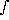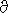# Weighting Functions

The outgoing radiance or upward radiance, Ru, for channel i from an atmospheric column is given by

Riu =Bi(T(p)) · (u(p)/ln p) d ln p

The weighting function is defined as

W(p)u(p)/ln p

and the contribution function is the integrand of the outgoing radiance calculation

C(p)Bi(T(p)) · (u(p)/ln p)

and can be normalized as

C(p)Bi(T(p))/Riu · (u(p)/ln p)

the information content will require derivative w.r.t. temperature of the radiation transfer equation and, therefore, the Kernel function represents the best estimate of the sounding level for temperature retrievals.

K(p)(Bi(T(p))/T) · (u(p)/ln p)